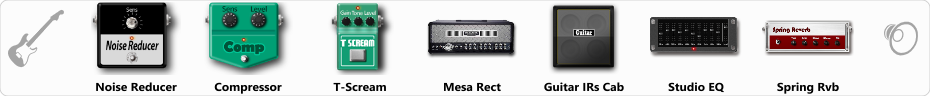# Mesa Boogie Dual Rectifier v2

Discussion in 'ToneLib-GFX presets' started by Serg10, Jul 26, 2019.

1. Mesa Boogie Dual Rectifier v2

Preset name: Mesa Boogie Dual Rectifier v2

Effects chain:Effect: "Noise Reducer" (Dynamics / Filter), active - "yes"
"Sens" = 82
"Mode" = Hard

Effect: "Compressor" (Dynamics / Filter), active - "yes"
"Sense" = 60
"Level" = 50

Effect: "T-Scream" (Overdrive / Distortion), active - "yes"
"Drive" = 43
"Tone" = 63
"Level" = 59

Effect: "Mesa Rect" (Amp simulators), active - "yes"
"Gain" = 81
"Bass" = 66
"Middle" = 36
"Treble" = 75
"Presence" = 80
"Master" = 60
"Output" = 50
"Level (dB)" = 0

Effect: "Guitar IRs Cab" (Cabinets), active - "yes"
"Model" = Mesa Rectifier (4x12")
"Mic Position" = Center
"Mic Distance" = Near
"Low Cut (Hz)" = 60
"Hi Cut (kHz)" = 20.0
"Mix" = 100
"Level (dB)" = 0

Effect: "Studio EQ" (Dynamics / Filter), active - "yes"
"31 Hz" = 0
"62 Hz" = 3
"125 Hz" = -3
"250 Hz" = -3
"500 Hz" = -2
"1 kHz" = 4
"2 kHz" = 2
"4 kHz" = 1
"8 kHz" = -3
"16 kHz" = 0
"above 16 kHz" = 0
"Level (dB)" = 0

Effect: "Spring Rvb" (Reverb), active - "yes"
"Time" = 7.0
"PreDelay" = 0
"LoDamp" = 16
"HiDamp" = 26
"Mix" = 28

Note: You will need to download and install the ToneLib-GFX software to use the preset.

#### Attached Files:

• ###### Mesa_Boogie_Dual_Rectifier_v2.tlgfx
File size:
641 bytes
Views:
9,283
Alejandro111, Summers, stek and 23 others like this.